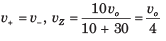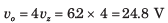Courses

# Test: Operational Amplifiers

## 20 Questions MCQ Test RRB JE for Electrical Engineering | Test: Operational Amplifiers

Description
This mock test of Test: Operational Amplifiers for Electronics and Communication Engineering (ECE) helps you for every Electronics and Communication Engineering (ECE) entrance exam. This contains 20 Multiple Choice Questions for Electronics and Communication Engineering (ECE) Test: Operational Amplifiers (mcq) to study with solutions a complete question bank. The solved questions answers in this Test: Operational Amplifiers quiz give you a good mix of easy questions and tough questions. Electronics and Communication Engineering (ECE) students definitely take this Test: Operational Amplifiers exercise for a better result in the exam. You can find other Test: Operational Amplifiers extra questions, long questions & short questions for Electronics and Communication Engineering (ECE) on EduRev as well by searching above.
QUESTION: 1

### For the circuit shown in fig. the input resistance is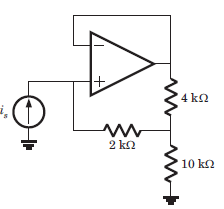Solution:

Since op-amp is ideal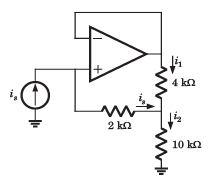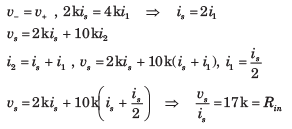QUESTION: 2

### In the circuit of fig. the op-amp slew rate is SR = 0.5 V/μs. If the amplitude of input signal is 0.02 V, then the maximum frequency that may be used is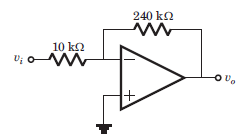Solution: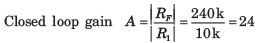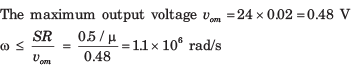QUESTION: 3

### In the circuit of fig. the input offset voltage and input offset current are Vio = 4 mV and Iio = 150 nA. The total output offset voltage is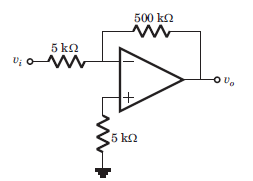Solution: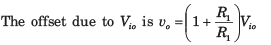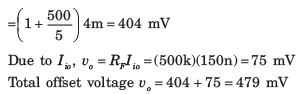QUESTION: 4

io = ?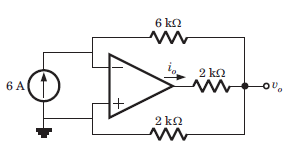Solution: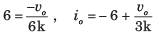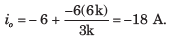QUESTION: 5

Consider the circuit shown below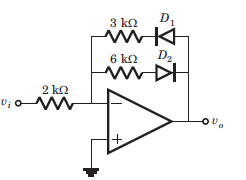Que: If vi =2 V, then output vo is

Solution:

If vi > 0, then v0 < 0, D1 blocks and D2 conducts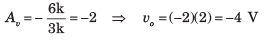QUESTION: 6

Consider the circuit shown belowQue: If vi = -2 V, then output vo is

Solution:

If vi < 0, then v0 > 0, D1 blocks and D2 conducts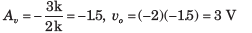QUESTION: 7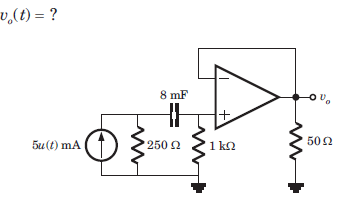Solution:

Voltage follower v0 = v = v+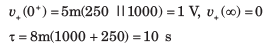QUESTION: 8

The circuit shown in fig. is at steady state before the switch opens at t = 0. The vc(t) for t > 0 is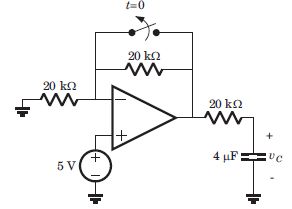Solution: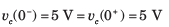For t > 0 the equivalent circuit is shown in fig.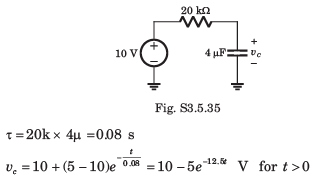QUESTION: 9

The LED in the circuit of fig. will be on if vis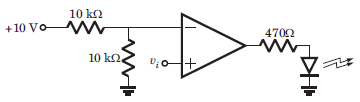Solution: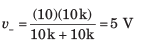When v+ > 5 V, output will be positive and LED will be on. Hence (C) is correct.

QUESTION: 10

In the circuit of fig. the CMRR of the op-amp is 60 dB. The magnitude of the vo is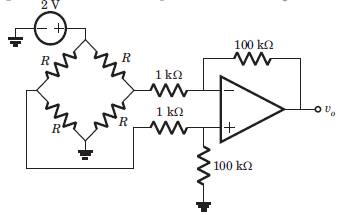Solution: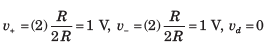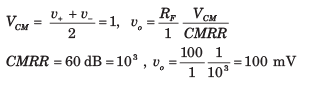QUESTION: 11

The analog multiplier X of fig. has the characteristics vp = v1v2 The output of this circuit is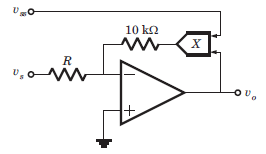Solution:

v+ = 0 = v-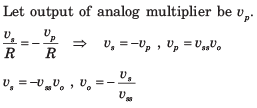QUESTION: 12

If the input to the ideal comparator shown in fig. is a sinusoidal signal of 8 V (peak to peak) without any DC component, then the output of the comparator has a duty cycle of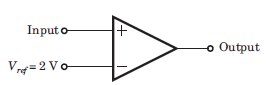Solution:

When vi > 2 V, output is positive. When vi < 2 V, output is negative.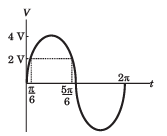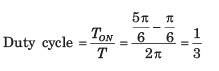QUESTION: 13

In the op-amp circuit given in fig. the load current iL is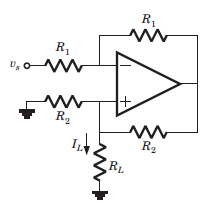Solution: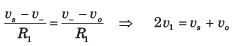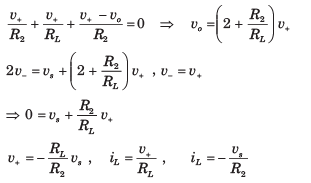QUESTION: 14

In the circuit of fig. output voltage is |vo| =1 V for a certain set of ω, R, an C. The |vo| will be 2 V if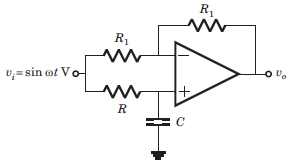Solution:

This is a all pass circuit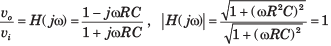Thus when ω and R is changed, the transfer function is unchanged

QUESTION: 15

In the circuit of fig. the 3 dB cutoff frequency is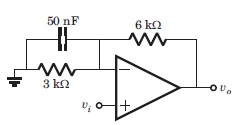Solution: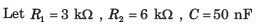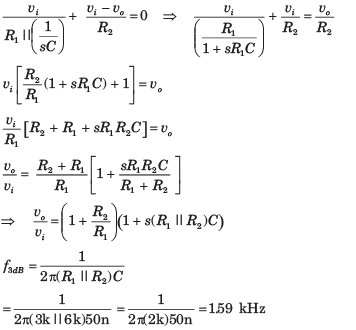QUESTION: 16

The phase shift oscillator of fig. operate at f = 80 kHz. The value of resistance RF is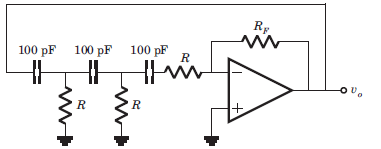Solution:

The oscillation frequency is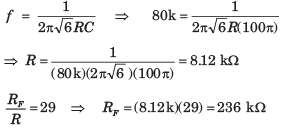QUESTION: 17

The value of C required for sinusoidal oscillation of frequency 1 kHz in the circuit of fig. is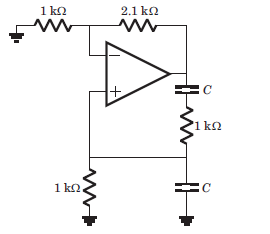Solution:

This is Wien-bridge oscillator. The ratio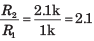is greater than 2. So there will be
oscillation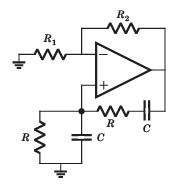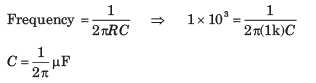QUESTION: 18

In the circuit shown in fig. the op-amp is ideal. If βF = 60, then the total current supplied by the 15 V source is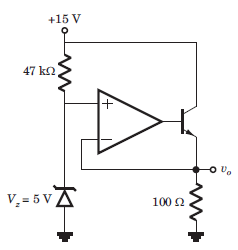Solution:

v+ = 5V = v = VE ,

The input current to the op-amp is zero.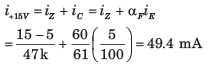QUESTION: 19

In the circuit in fig. both transistor Q1 and Q2 are identical. The output voltage at T = 300 K is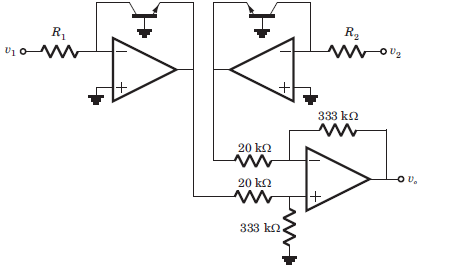Solution: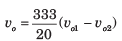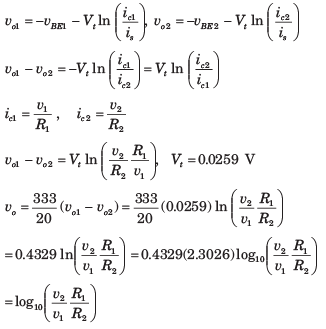QUESTION: 20

In the op-amp series regulator circuit of fig. Vz = 62. V, VBE = 0.7 V and β = 60. The output voltage vo is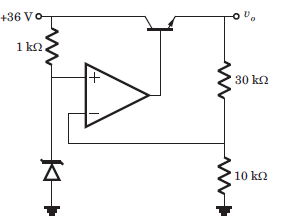Solution: